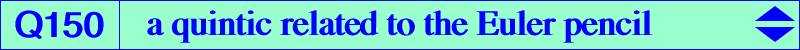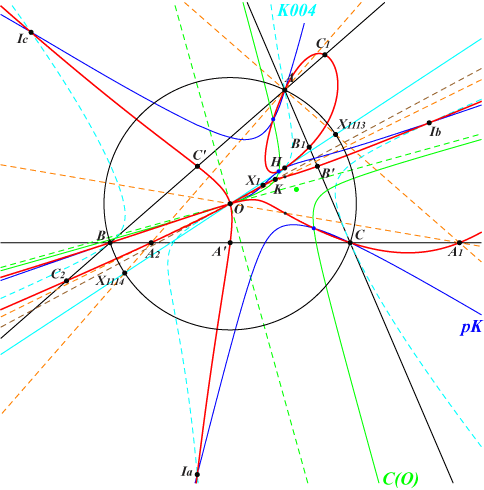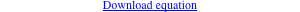too complicated to be written here. Click on the link to download a text file.X(1), X(3), X(4), X(6), X(2574), X(2575) excenters midpoints of ABC vertices of the cevian triangles of X(1113) and X(1114) infinite points of the altitudes of ABC other points belowTable 27 contains the cubics of the Euler pencil. These are the isogonal pKs with pivot P on the Euler line. Any pK(X6, P) passes through the circum-center O and the polar conic C(O) of O passes through O, K, X(2574), X(2575). See further details in Table 27. When P traverses the Euler line, a pK(X6, P) meets its corresponding polar conic C(O) at six points (one being O itself counted twice) and then the locus of these points is the quintic Q150. See the related Q151. Now denote by P1 and P2 any two distinct points on the Euler line, pK1 and pK2 the corresponding pivotal cubics, C1(O) and C2(O) the polar conics of O. The quintic may be written under the form Q150 =pK1 x C2(O) – pK2 x C1(O). Properties • O is a triple point on Q150 with tangents the Brocard axis and the parallels at O to the asymptotes of the Jerabek hyperbola. • The tangents at A, B, C, X(4), the in/excenters all concur at O. • Q150 is invariant under the Cundy-Parry involution Φ studied in CL037. A line passing through O meets Q150 at O (counting for three) and two other points lying on a same rectangular circum-hyperbola. • In particular, the Φ–images of the vertices A1, B1, C1 and A2, B2, C2 of the cevian triangles of X(1113) and X(1114) are the six remaining points of Q150 on the altitudes of ABC. In other words, if A1 = BC /\ AX(1113) then AH /\ A1O lies on Q150, the five other points similarly.